Скачать презентацию Hodgkin-Huxley Model and Fitz Hugh-Nagumo Model Nervous

• Количество слайдов: 16Hodgkin-Huxley Model and Fitz. Hugh-Nagumo Model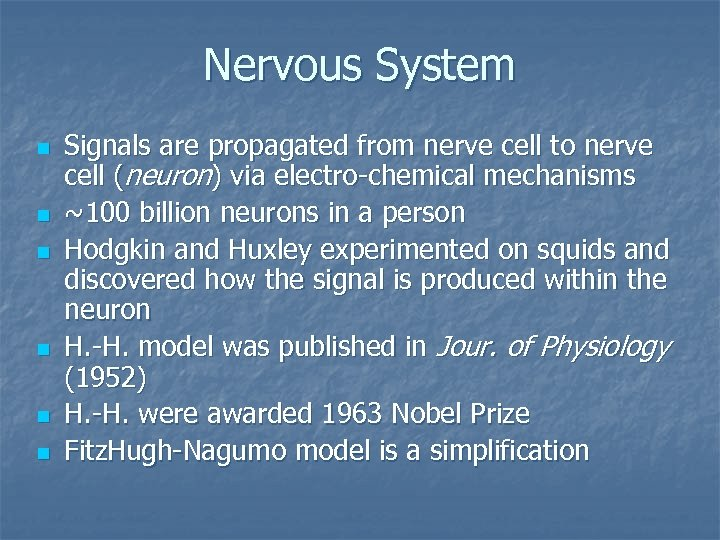Nervous System n n n Signals are propagated from nerve cell to nerve cell (neuron) via electro-chemical mechanisms ~100 billion neurons in a person Hodgkin and Huxley experimented on squids and discovered how the signal is produced within the neuron H. -H. model was published in Jour. of Physiology (1952) H. -H. were awarded 1963 Nobel Prize Fitz. Hugh-Nagumo model is a simplification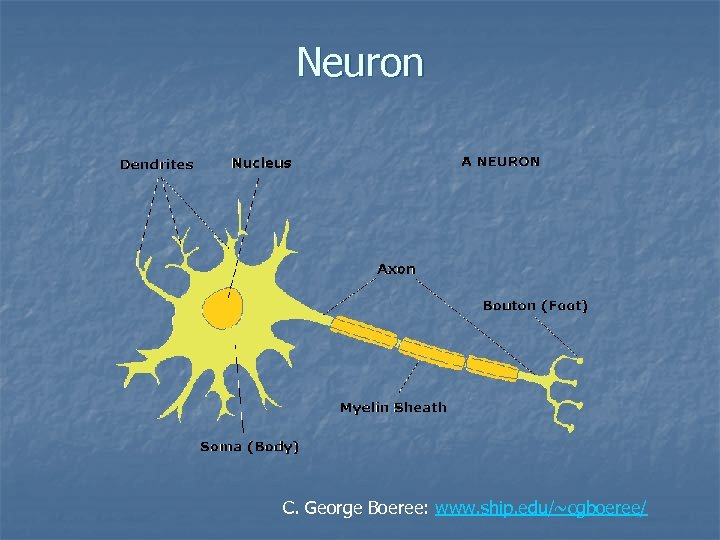Neuron C. George Boeree: www. ship. edu/~cgboeree/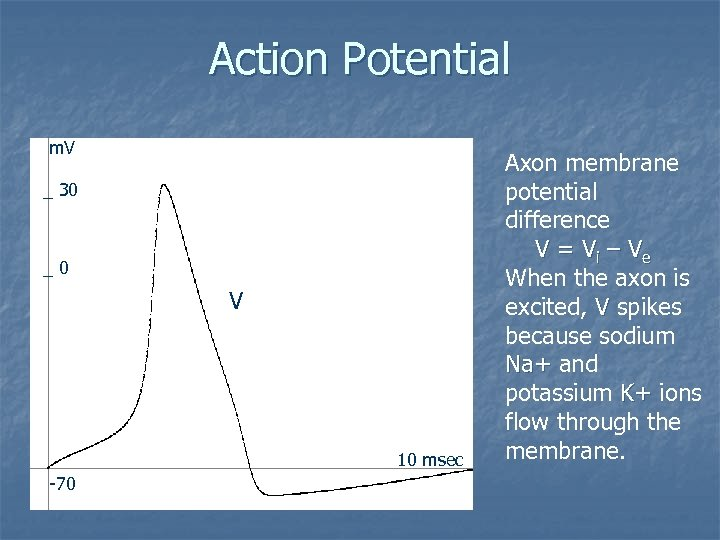Action Potential m. V _ 30 _0 V 10 msec -70 Axon membrane potential difference V = V i – Ve When the axon is excited, V spikes because sodium Na+ and potassium K+ ions flow through the membrane.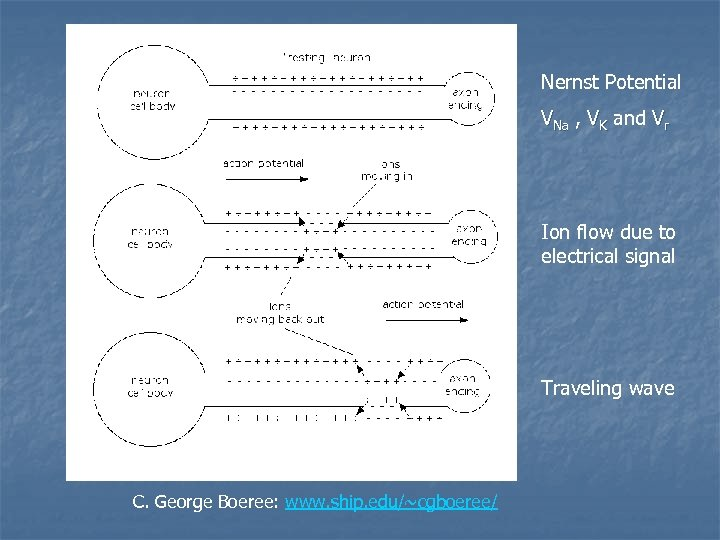Nernst Potential VNa , VK and Vr Ion flow due to electrical signal Traveling wave C. George Boeree: www. ship. edu/~cgboeree/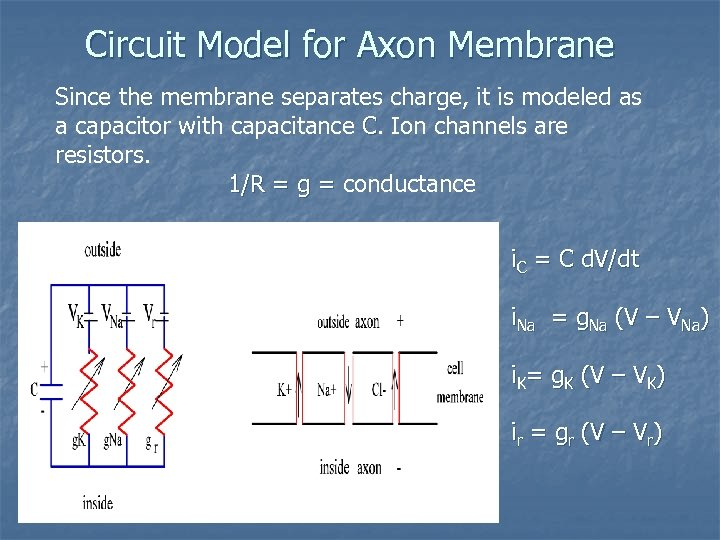Circuit Model for Axon Membrane Since the membrane separates charge, it is modeled as a capacitor with capacitance C. Ion channels are resistors. 1/R = g = conductance i. C = C d. V/dt i. Na = g. Na (V – VNa) i. K= g. K (V – VK) ir = gr (V – Vr)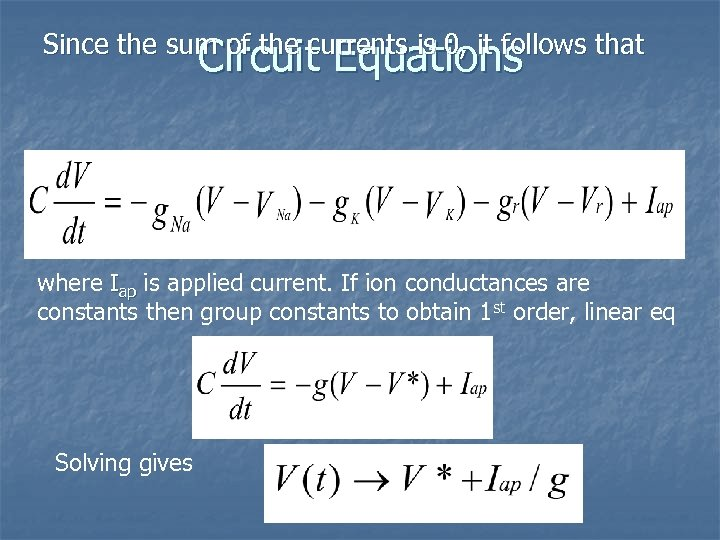Since the sum of the currents is 0, it follows that Circuit Equations where Iap is applied current. If ion conductances are constants then group constants to obtain 1 st order, linear eq Solving gives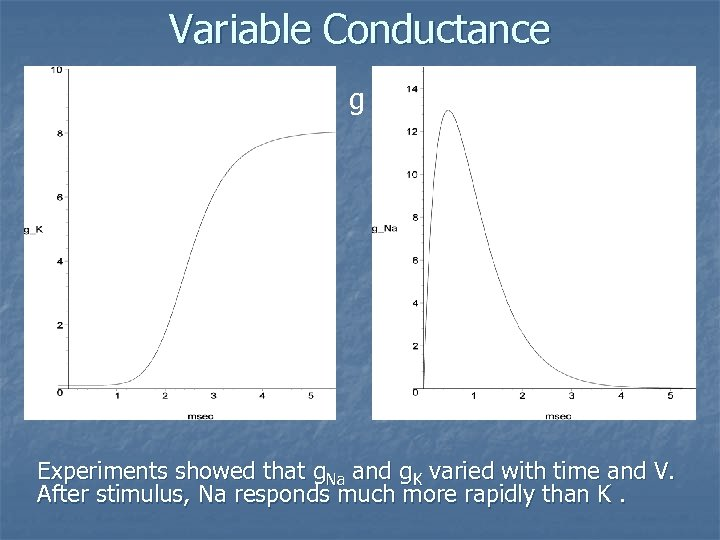Variable Conductance g Experiments showed that g. Na and g. K varied with time and V. After stimulus, Na responds much more rapidly than K.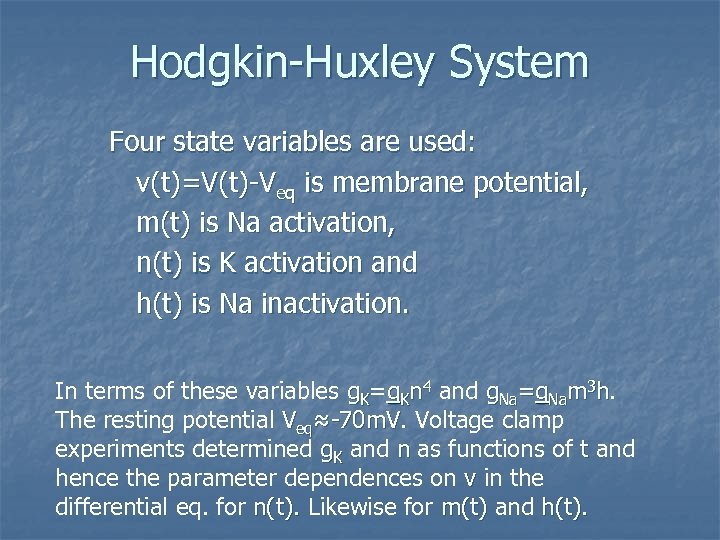Hodgkin-Huxley System Four state variables are used: v(t)=V(t)-Veq is membrane potential, m(t) is Na activation, n(t) is K activation and h(t) is Na inactivation. In terms of these variables g. K=g. Kn 4 and g. Na=g. Nam 3 h. The resting potential Veq≈-70 m. V. Voltage clamp ≈-70 m. V experiments determined g. K and n as functions of t and hence the parameter dependences on v in the differential eq. for n(t). Likewise for m(t) and h(t).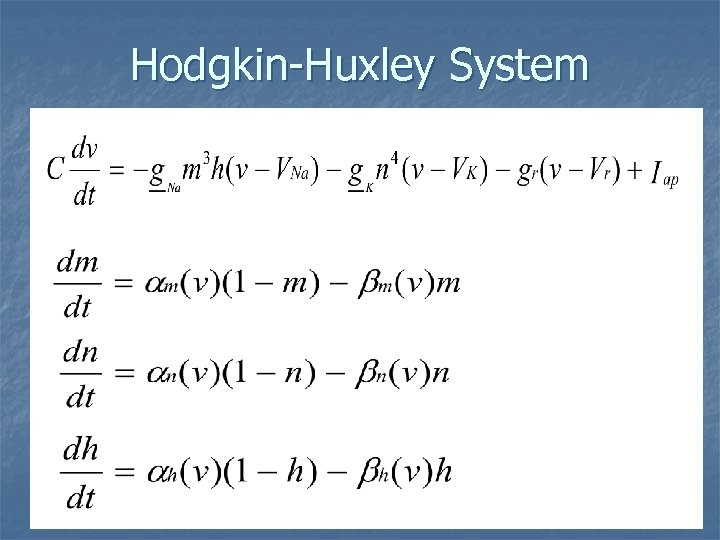Hodgkin-Huxley System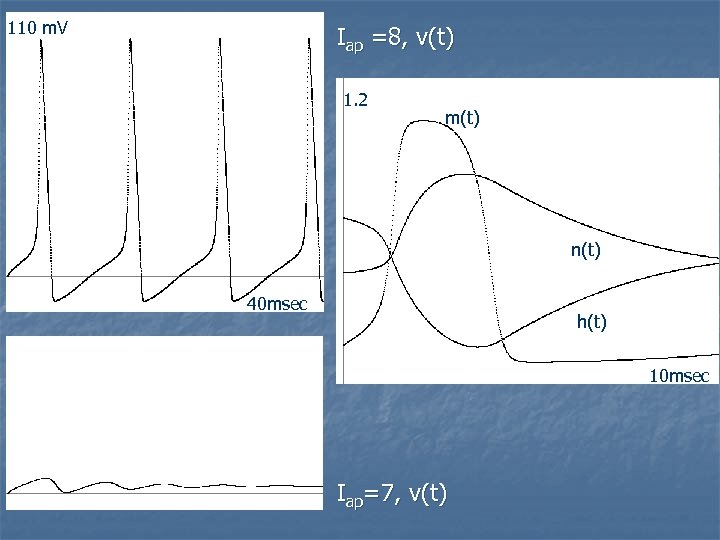110 m. V Iap =8, v(t) 1. 2 m(t) n(t) 40 msec h(t) 10 msec Iap=7, v(t)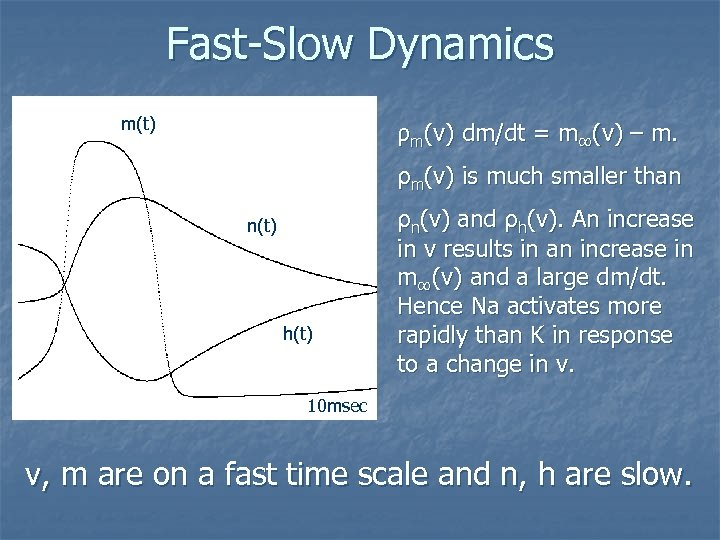Fast-Slow Dynamics m(t) ρm(v) dm/dt = m∞(v) – m. ρm(v) is much smaller than n(t) h(t) ρn(v) and ρh(v). An increase in v results in an increase in m∞(v) and a large dm/dt. Hence Na activates more rapidly than K in response to a change in v. 10 msec v, m are on a fast time scale and n, h are slow.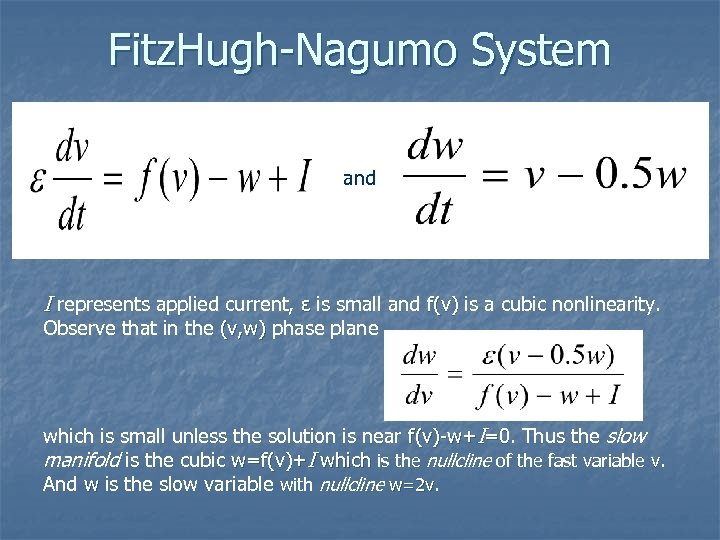Fitz. Hugh-Nagumo System and I represents applied current, ε is small and f(v) is a cubic nonlinearity. Observe that in the (v, w) phase plane which is small unless the solution is near f(v)-w+I=0. Thus the slow =0 manifold is the cubic w=f(v)+I which is the nullcline of the fast variable v. And w is the slow variable with nullcline w=2 v.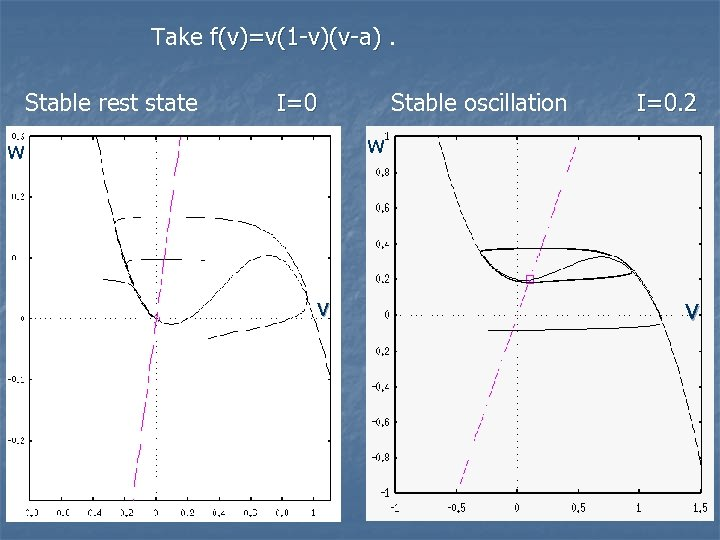Take f(v)=v(1 -v)(v-a). Stable rest state I=0 Stable oscillation I=0. 2 w w v v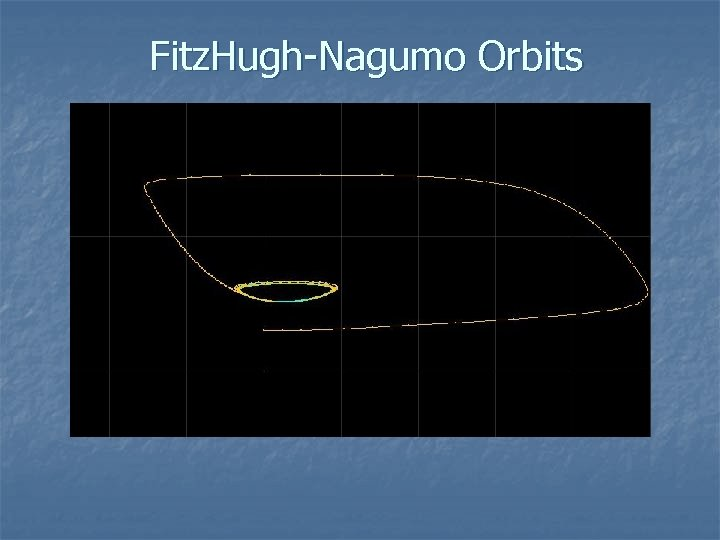Fitz. Hugh-Nagumo Orbits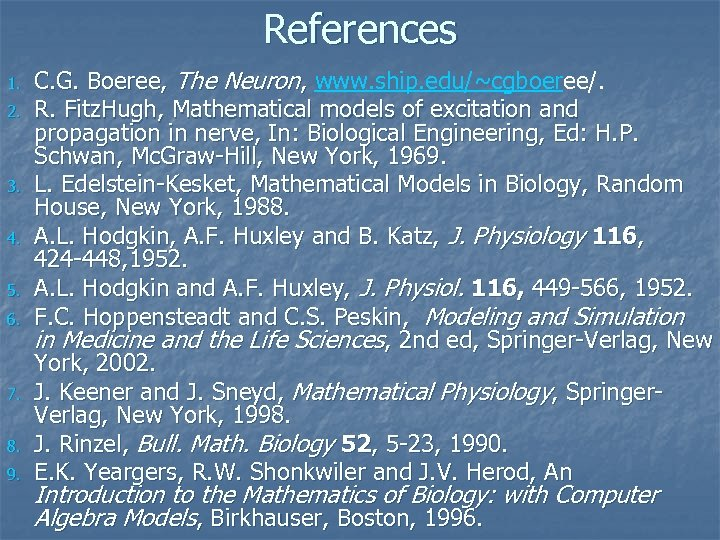References 1. 2. 3. 4. 5. 6. 7. 8. 9. C. G. Boeree, The Neuron, www. ship. edu/~cgboeree/. R. Fitz. Hugh, Mathematical models of excitation and propagation in nerve, In: Biological Engineering, Ed: H. P. Schwan, Mc. Graw-Hill, New York, 1969. L. Edelstein-Kesket, Mathematical Models in Biology, Random House, New York, 1988. A. L. Hodgkin, A. F. Huxley and B. Katz, J. Physiology 116, 424 -448, 1952. A. L. Hodgkin and A. F. Huxley, J. Physiol. 116, 449 -566, 1952. F. C. Hoppensteadt and C. S. Peskin, Modeling and Simulation in Medicine and the Life Sciences, 2 nd ed, Springer-Verlag, New York, 2002. J. Keener and J. Sneyd, Mathematical Physiology, Springer. Verlag, New York, 1998. J. Rinzel, Bull. Math. Biology 52, 5 -23, 1990. E. K. Yeargers, R. W. Shonkwiler and J. V. Herod, An Introduction to the Mathematics of Biology: with Computer Algebra Models, Birkhauser, Boston, 1996.